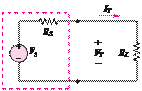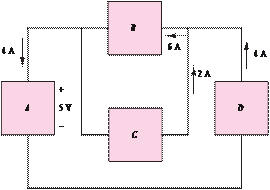### Create an Account

Already have account?

### Forgot Your Password ?

Home / Questions / For the circuit shown in Figure P224 determine which components are supplying power and w...

# For the circuit shown in Figure P224 determine which components are supplying power and which are dissipating power Also determine the amount of power dissipated and suppliedFor the circuit shown in Figure P2.24, determine which components are supplying power and which are dissipating power. Also determine the amount of power dissipated and supplied.

+     2 V   _Nonideal source

Figure P2.28

2.29      A GE SoftWhite Longlife lightbulb is rated as follows:

+                PR  = rated power  = 60 W

3 V            POR = rated optical power = 820 lumens (lm) (average)

 =        W

_                1 lumen

1     680

Figure P2.24

Operating life = 1,500 h (average)

VR  = rated operating voltage  = 115 V

The resistance of the filament of the bulb, measured with a standard multimeter, is 16.7 Q. When the bulb

is connected into a circuit and is operating at the rated values given above, determine

a.    The resistance of the filament.

b.    The efficiency of the bulb.

Jun 19 2020 View more View Less

#### Answer (Solved)Subscribe To Get Solution